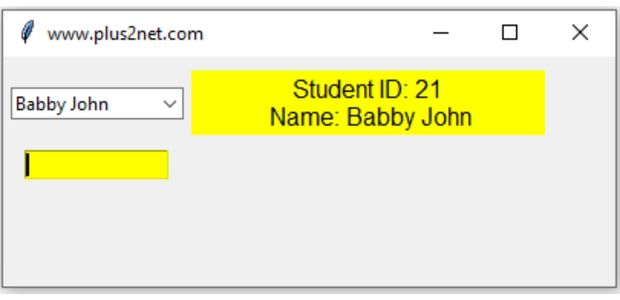# Searching Combobox optionsFrom our student table we will collect student name and student id by searching the name column based on the user char entry.

As the user enters chars in the Combobox, we will search the table by using LIKE query and return the matching results as options.

Searching options of the Combobox from MySQL database table as source

We will create a dictionary by using returned names from the database. Here student id will be the key and names will be used as values of the dictionary. Inititally we will populate the Combobox with all the records as returned by table ( without any filter )
``````import tkinter as tk
from tkinter import ttk

my_w = tk.Tk()
my_w.geometry("400x150")  # Size of the window
from sqlalchemy import create_engine
from sqlalchemy.sql import text

query = "SELECT id,name FROM student" # SQL
my_data = list(my_conn.execute(query))
my_dict = {}

p_id, p_name = 0, ""
for row in my_data:
my_dict[row] = row``````
Using the above dictionary my_dict, values we will populate in the Combobox cb1, check the values parameter.
``````sel = tk.StringVar()  # string variable
cb1 = ttk.Combobox(my_w, values=list(my_dict.values()),
width=15, textvariable=sel)
As we can use Combobox as entry widget by entering data other than the given options ( that’s why the name Combobox ) , we will trigger a function my_upd() using the trace method of StringVar() sel.
``sel.trace("w", my_upd) # trigger the function on change of sel``
Inside the function my_upd() we will create the SQL and get the records from table. Note that while creating the query we will use the user input by taking cb1.get() value.
``````def my_upd(*args):
my_dict = {}
query = text("SELECT id,name FROM student WHERE name like :e1")
#print(query)
my_data = list(my_conn.execute(query, e1="%" + cb1.get() + "%"))
for row in my_data:
my_dict[row] = row
#print(my_data)
cb1["values"] = [] # remove all previous options
cb1["values"] = list(my_dict.values()) # add new options
l1.config(text="Number records : " + str(len(my_data)))``````
Based on the string what the user has entered we are displaying the number of records found using our Label l1. Once the user select one of the option and moves out ( FocusOut ) , we will trigger the my_disp() function.
``````def my_disp(*args):
global p_id, p_name
l1.config(text="") # remove the text from Label
for i, j in my_dict.items(): # all key and values of the dictionary
if j == sel.get(): # matching value
p_id, p_name = i, j # set key and value
l1.config(text=" Student ID: " + str(i) + " \n Name: " + j)
break # come out of the for loop ``````
``````import tkinter as tk
from tkinter import ttk

my_w = tk.Tk()
my_w.geometry("400x150")  # Size of the window
from sqlalchemy import create_engine
from sqlalchemy.sql import text

my_conn = create_engine("mysql+mysqldb://id:pw@localhost/my_db")
query = "SELECT id,name FROM student"
my_data = list(my_conn.execute(query))
my_dict = {}

p_id, p_name = 0, ""
for row in my_data:
my_dict[row] = row

def my_upd(*args):
my_dict = {}
query = text("SELECT id,name FROM student WHERE name like :e1")
#print(query)
my_data = list(my_conn.execute(query, e1="%" + cb1.get() + "%"))
for row in my_data:
my_dict[row] = row
#print(my_data)
cb1["values"] = [] # remove all previous options
cb1["values"] = list(my_dict.values()) # add new options
l1.config(text="Number records : " + str(len(my_data)))

def my_disp(*args):
global p_id, p_name
l1.config(text="") # remove the text from Label
for i, j in my_dict.items(): # all key and values of the dictionary
if j == sel.get(): # matching value
p_id, p_name = i, j # set key and value
l1.config(text=" Student ID: " + str(i) + " \n Name: " + j)
break # come out of the for loop

sel = tk.StringVar()  # string variable
cb1 = ttk.Combobox(my_w, values=list(my_dict.values()),textvariable=sel)
l1 = tk.Label(my_w, text="Result", bg="yellow", width=25,font=20)
l1.grid(row=1, column=2)
e1 = tk.Entry(my_w, text="Result", bg="yellow", width=15)
e1.grid(row=2, column=1)
sel.trace("w", my_upd) # trigger the function on change of sel
cb1.bind("<FocusOut>", my_disp) # once focus is out of the Combobox
my_w.mainloop()  # Keep the window open
``````

Subscribe to our YouTube Channel here

## Subscribe

* indicates required
Subscribe to plus2netplus2net.com